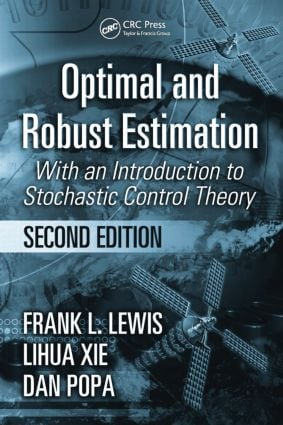Optimal and Robust Estimation

With an Introduction to Stochastic Control Theory, Second Edition, 2nd Edition

CRC Press

552 pages | 125 B/W Illus.

Purchasing Options:\$ = USD
Hardback: 9780849390081
pub: 2007-09-17
SAVE ~\$35.00
\$175.00
\$140.00
x
eBook (VitalSource) : 9781315221656
pub: 2017-12-19
from \$105.00

FREE Standard Shipping!

Description

More than a decade ago, world-renowned control systems authority Frank L. Lewis introduced what would become a standard textbook on estimation, under the title Optimal Estimation, used in top universities throughout the world. The time has come for a new edition of this classic text, and Lewis enlisted the aid of two accomplished experts to bring the book completely up to date with the estimation methods driving today's high-performance systems.

A Classic Revisited

Optimal and Robust Estimation: With an Introduction to Stochastic Control Theory, Second Edition reflects new developments in estimation theory and design techniques. As the title suggests, the major feature of this edition is the inclusion of robust methods. Three new chapters cover the robust Kalman filter, H-infinity filtering, and H-infinity filtering of discrete-time systems.

Modern Tools for Tomorrow's Engineers

This text overflows with examples that highlight practical applications of the theory and concepts. Design algorithms appear conveniently in tables, allowing students quick reference, easy implementation into software, and intuitive comparisons for selecting the best algorithm for a given application. In addition, downloadable MATLAB® code allows students to gain hands-on experience with industry-standard software tools for a wide variety of applications.

This cutting-edge and highly interactive text makes teaching, and learning, estimation methods easier and more modern than ever.

OPTIMAL ESTIMATION

Classical Estimation Theory

Mean-Square Estimation

Maximum-Likelihood Estimation

The Cramer-Rao Bound

Recursive Estimation

Wiener Filtering

Problems

Discrete-Time Kalman Filter

Deterministic State Observer

Linear Stochastic Systems

The Discrete-Time Kalman Filter

Discrete Measurements of Continuous-Time Systems

Error Dynamics and Statistical Steady State

Frequency Domain Results

Correlated Noise and Shaping Filters

Optimal Smoothing

Problems

Continuous-Time Kalman Filter

Derivation from Discrete Kalman Filter

Some Examples

Derivation from Wiener-Hopf Equation

Error Dynamics and Statistical Steady State

Frequency Domain Results

Correlated Noise and Shaping Filters

Discrete Measurements of Continuous-Time Systems

Optimal Smoothing

Problems

Kalman Filter Design and Implementation

Modeling Errors, Divergence, and Exponential Data Weighting

Reduced-Order Filters and Decoupling

Using Suboptimal Gains

Scalar Measurement Updating

Problems

Estimation for Nonlinear Systems

Update of the Hyperstate

General Update of Mean and Covariance

Extended Kalman Filter

Application to Robotics and Adaptive Sampling

Problems

ROBUST ESTIMATION

Robust Kalman Filter

Systems with Modeling Uncertainties

Robust Finite Horizon Kalman A Priori Filter

Robust Stationary Kalman A Priori Filter

Convergence Analysis

Linear Matrix Inequality Approach

Robust Kalman Filtering for Continuous-Time Systems

Problems

H-Infinity Filtering of Continuous-Time Systems

H-Infinity Filtering Problem

Finite Horizon H-Infinity Linear Filter

Characterization of All Finite Horizon H-Infinity Linear Filters

Stationary H-Infinity Filter-Riccati Equation Approach

Relationship with the Kalman Filter

Convergence Analysis

H-Infinity Filtering for a Special Class of Signal Models

Stationary H-Infinity Filter-Linear Matrix Inequality Approach

Problems

H-Infinity Filtering of Discrete-Time Systems

Discrete-Time H-Infinity Filtering Problem

H-Infinity A Priori Filter

H-Infinity A Posteriori Filter

Polynomial Approach to H-Infinity Estimation

J-Spectral Factorization

Applications in Channel Equalization

Problems

OPTIMAL STOCHASTIC CONTROL

Stochastic Control for State Variable Systems

Dynamic Programming Approach

Continuous-Time Linear Quadratic Gaussian Problem

Discrete-Time Linear Quadratic Gaussian Problem

Problems

Stochastic Control for Polynomial Systems

Polynomial Representation of Stochastic Systems

Optimal Prediction

Minimum Variance Control

Polynomial Linear Quadratic Gaussian Regulator

Problems

Appendix A: Review of Matrix Algebra

Basic Definitions and Facts

Partitioned Matrices

Quadratic Forms and Definiteness

Matrix Calculus

References

Index